# getdist.plots¶

This module is used for making plots from samples. The `get_single_plotter()` and `get_subplot_plotter()` functions are used to make a plotter instance, which is then used to make and export plots.

Many plotter functions take a roots argument, which is either a root name for some chain files, or an in-memory `MCSamples` instance. You can also make comparison plots by giving a list of either of these.

Parameter are referenced simply by name (as specified in the .paramnames file when loading from file, or set in the `MCSamples` instance). For functions that takes lists of parameters, these can be just lists of names. You can also use glob patterns to match specific subsets of parameters (e.g. x* to match all parameters with names starting with x).

 `get_single_plotter` Get a `GetDistPlotter` for making a single plot of fixed width. `get_subplot_plotter` Get a `GetDistPlotter` for making an array of subplots. `GetDistPlotter` Main class for making plots from one or more sets of samples. `GetDistPlotSettings` Settings class (colors, sizes, font, styles etc.)
exception `getdist.plots.``GetDistPlotError`[source]

An exception that is raised when there is an error plotting

class `getdist.plots.``GetDistPlotSettings`(subplot_size_inch: float = 2, fig_width_inch: Optional[float] = None)[source]

Settings class (colors, sizes, font, styles etc.)

Variables: alpha_factor_contour_lines – alpha factor for adding contour lines between filled contours alpha_filled_add – alpha for adding filled contours to a plot axes_fontsize – Size for axis font at reference axis size axes_labelsize – Size for axis label font at reference axis size axis_marker_color – The color for a marker axis_marker_ls – The line style for a marker axis_marker_lw – The line width for a marker axis_tick_powerlimits – exponents at which to use scientific notation for axis tick labels axis_tick_max_labels – maximum number of tick labels per axis axis_tick_step_groups – steps to try for axis ticks, in grouped in order of preference axis_tick_x_rotation – The rotation for the x tick label in degrees axis_tick_y_rotation – The rotation for the y tick label in degrees colorbar_axes_fontsize – size for tick labels on colorbar (None for default to match axes font size) colorbar_label_pad – padding for the colorbar label colorbar_label_rotation – angle to rotate colorbar label (set to zero if -90 default gives layout problem) colorbar_tick_rotation – angle to rotate colorbar tick labels colormap – a Matplotlib color map for shading colormap_scatter – a Matplotlib color map for 3D scatter plots constrained_layout – use matplotlib’s constrained-layout to fit plots within the figure and avoid overlaps. fig_width_inch – The width of the figure in inches figure_legend_frame – draw box around figure legend figure_legend_loc – The location for the figure legend figure_legend_ncol – number of columns for figure legend (set to zero to use defaults) fontsize – font size for text (and ultimate fallback when others not set) legend_colored_text – use colored text for legend labels rather than separate color blocks legend_fontsize – The font size for the legend (defaults to fontsize) legend_frac_subplot_margin – fraction of subplot size to use for spacing figure legend above plots legend_frame – draw box around legend legend_loc – The location for the legend legend_rect_border – whether to have black border around solid color boxes in legends line_dash_styles – dict mapping line styles to detailed dash styles, default: {’–’: (3, 2), ‘-.’: (4, 1, 1, 1)} line_labels – True if you want to automatically add legends when adding more than one line to subplots line_styles – list of default line styles/colors ([‘-k’, ‘-r’, ‘–C0’, …]) or name of a standard colormap (e.g. tab10), or a list of tuples of line styles and colors for each line linewidth – relative linewidth (at reference size) linewidth_contour – linewidth for lines in filled contours linewidth_meanlikes – linewidth for mean likelihood lines no_triangle_axis_labels – whether subplots in triangle plots should show axis labels if not at the edge norm_1d_density – whether to normolize 1D densities (otherwise normalized to unit peak value) norm_prob_label – label for the y axis in normalized 1D density plots num_plot_contours – number of contours to plot in 2D plots (up to number of contours in analysis settings) num_shades – number of distinct colors to use for shading shaded 2D plots param_names_for_labels – file name of .paramnames file to use for overriding parameter labels for plotting plot_args – dict, or list of dicts, giving settings like color, ls, alpha, etc. to apply for a plot or each line added plot_meanlikes – include mean likelihood lines in 1D plots prob_label – label for the y axis in unnormalized 1D density plots prob_y_ticks – show ticks on y axis for 1D density plots progress – write out some status scaling – True to scale down fonts and lines for smaller subplots; False to use fixed sizes. scaling_max_axis_size – font sizes will only be scaled for subplot widths (in inches) smaller than this. scaling_factor – factor by which to multiply the difference of the axis size to the reference size when scaling font sizes scaling_reference_size – axis width (in inches) at which font sizes are specified. direct_scaling – True to directly scale the font size with the axis size for small axes (can be very small) scatter_size – size of points in “3D” scatter plots shade_level_scale – shading contour colors are put at [0:1:spacing]**shade_level_scale shade_meanlikes – 2D shading uses mean likelihoods rather than marginalized density solid_colors – List of default colors for filled 2D plots or the name of a colormap (e.g. tab10). If a list, each element is either a color, or a tuple of values for different contour levels. solid_contour_palefactor – factor by which to make 2D outer filled contours paler when only specifying one contour color subplot_size_ratio – ratio of width and height of subplots tight_layout – use tight_layout to layout, avoid overlaps and remove white space; if it doesn’t work try constrained_layout. If true it is applied when calling `finish_plot()` (which is called automatically by plots_xd(), triangle_plot and rectangle_plot). title_limit – show parameter limits over 1D plots, 1 for first limit (68% default), 2 second, etc. title_limit_labels – whether or not to include parameter label when adding limits above 1D plots title_limit_fontsize – font size to use for limits in plot titles (defaults to axes_labelsize)

If fig_width_inch set, fixed setting for fixed total figure size in inches. Otherwise use subplot_size_inch to determine default font sizes etc., and figure will then be as wide as necessary to show all subplots at specified size.

Parameters: subplot_size_inch – Determines the size of subplots, and hence default font sizes fig_width_inch – The width of the figure in inches, If set, forces fixed total size.
`rc_sizes`(axes_fontsize=None, lab_fontsize=None, legend_fontsize=None)[source]

Sets the font sizes by default from matplotlib.rcParams defaults

Parameters: axes_fontsize – The font size for the plot axes tick labels (default: xtick.labelsize). lab_fontsize – The font size for the plot’s axis labels (default: axes.labelsize) legend_fontsize – The font size for the plot’s legend (default: legend.fontsize)
`set_with_subplot_size`(size_inch=3.5, size_mm=None, size_ratio=None)[source]

Sets the subplot’s size, either in inches or in millimeters. If both are set, uses millimeters.

Parameters: size_inch – The size to set in inches; is ignored if size_mm is set. size_mm – None if not used, otherwise the size in millimeters we want to set for the subplot. size_ratio – ratio of height to width of subplots
class `getdist.plots.``GetDistPlotter`(chain_dir: Union[str, Iterable[str], None] = None, settings: Optional[getdist.plots.GetDistPlotSettings] = None, analysis_settings: Union[str, dict, getdist.inifile.IniFile] = None, auto_close=False)[source]

Main class for making plots from one or more sets of samples.

Variables: settings – a `GetDistPlotSettings` instance with settings subplots – a 2D array of `Axes` for subplots sample_analyser – a `MCSampleAnalysis` instance for getting `MCSamples` and derived data from a given root name tag (e.g. sample_analyser.samples_for_root(‘rootname’)) chain_dir – Set this to a directory or grid directory hierarchy to search for chains (can also be a list of such, searched in order) analysis_settings – The settings to be used by `MCSampleAnalysis` when analysing samples auto_close – whether to automatically close the figure whenever a new plot made or this instance released
`add_1d`(root, param, plotno=0, normalized=None, ax=None, title_limit=None, **kwargs)[source]

Low-level function to add a 1D marginalized density line to a plot

Parameters: root – The root name of the samples param – The parameter name plotno – The index of the line being added to the plot normalized – True if areas under lines should match, False if normalized to unit maximum. Default from settings.norm_1d_density. ax – optional `Axes` instance (or y,x subplot coordinate) to add to (defaults to current plot or the first/main plot if none) title_limit – if not None, a maginalized limit (1,2..) to print as the title of the plot kwargs – arguments for `plot()` min, max for the plotted density
`add_2d_contours`(root, param1=None, param2=None, plotno=0, of=None, cols=None, contour_levels=None, add_legend_proxy=True, param_pair=None, density=None, alpha=None, ax=None, **kwargs)[source]

Low-level function to add 2D contours to plot for samples with given root name and parameters

Parameters: root – The root name of samples to use or a MixtureND gaussian mixture param1 – x parameter param2 – y parameter plotno – The index of the contour lines being added of – the total number of contours being added (this is line plotno of of) cols – optional list of colors to use for contours, by default uses default for this plotno contour_levels – levels at which to plot the contours, by default given by contours array in the analysis settings add_legend_proxy – True if should add a proxy to the legend of this plot. param_pair – an [x,y] parameter name pair if you prefer to provide this rather than param1 and param2 density – optional `Density2D` to plot rather than that computed automatically from the samples alpha – alpha for the contours added ax – optional `Axes` instance (or y,x subplot coordinate) to add to (defaults to current plot or the first/main plot if none) kwargs – optional keyword arguments: filled: True to make filled contours color: top color to automatically make paling contour colours for a filled plot kwargs for `contour()` and `contourf()` bounds (from `bounds()`) for the 2D density plotted
`add_2d_covariance`(means, cov, xvals=None, yvals=None, def_width=4.0, samples_per_std=50.0, **kwargs)[source]

Plot 2D Gaussian ellipse. By default plots contours for 1 and 2 sigma. Specify contour_levels argument to plot other contours (for density normalized to peak at unity).

Parameters: means – array of y cov – the 2x2 covariance xvals – optional array of x values to evaluate at yvals – optional array of y values to evaluate at def_width – if evaluation array not specified, width to use in units of standard deviation samples_per_std – if evaluation array not specified, number of grid points per standard deviation kwargs – keyword arguments for `add_2D_contours()`
`add_2d_density_contours`(density, **kwargs)[source]

Low-level function to add 2D contours to a plot using provided density

Parameters: density – a `densities.Density2D` instance kwargs – arguments for `add_2d_contours()` bounds (from `bounds()`) of density
`add_2d_scatter`(root, x, y, color='k', alpha=1, extra_thin=1, scatter_size=None, ax=None)[source]

Low-level function to adds a 2D sample scatter plot to the current axes (or ax if specified).

Parameters: root – The root name of the samples to use x – name of x parameter y – name of y parameter color – color to plot the samples alpha – The alpha to use. extra_thin – thin the weight one samples by this additional factor before plotting scatter_size – point size (default: settings.scatter_size) ax – optional `Axes` instance (or y,x subplot coordinate) to add to (defaults to current plot or the first/main plot if none) (xmin, xmax), (ymin, ymax) bounds for the axes.
`add_2d_shading`(root, param1, param2, colormap=None, density=None, ax=None, **kwargs)[source]

Parameters: root – The root name of samples to use param1 – x parameter param2 – y parameter colormap – color map, default to settings.colormap (see `GetDistPlotSettings`) density – optional user-provided `Density2D` to plot rather than the auto-generated density from the samples ax – optional `Axes` instance (or y,x subplot coordinate) to add to (defaults to current plot or the first/main plot if none) kwargs – keyword arguments for `contourf()`
`add_3d_scatter`(root, params, color_bar=True, alpha=1, extra_thin=1, scatter_size=None, ax=None, alpha_samples=False, **kwargs)[source]

Low-level function to add a 3D scatter plot to the current axes (or ax if specified). Here 3D means a 2D plot, with samples colored by a third parameter.

Parameters: root – The root name of the samples to use params – list of parameters to plot color_bar – True to add a colorbar for the plotted scatter color alpha – The alpha to use. extra_thin – thin the weight one samples by this additional factor before plotting scatter_size – point size (default: settings.scatter_size) alpha_samples – use all samples, giving each point alpha corresponding to relative weight ax – optional `Axes` instance (or y,x subplot coordinate) to add to (defaults to current plot or the first/main plot if none) kwargs – arguments for `add_colorbar()` (xmin, xmax), (ymin, ymax) bounds for the axes.
`add_bands`(x, y, errors, color='gray', nbands=2, alphas=(0.25, 0.15, 0.1), lw=0.2, lw_center=None, linecolor='k', ax=None)[source]

Add a constraint band as a function of x showing e.g. a 1 and 2 sigma range.

Parameters: x – array of x values y – array of central values for the band as function of x errors – array of errors as a function of x color – a fill color nbands – number of bands to plot. If errors are 1 sigma, using nbands=2 will plot 1 and 2 sigma. alphas – tuple of alpha factors to use for each error band lw – linewidth for the edges of the bands lw_center – linewidth for the central mean line (zero or None not to have one, the default) linecolor – a line color for central line ax – optional `Axes` instance (or y,x subplot coordinate) to add to (defaults to current plot or the first/main plot if none)
`add_colorbar`(param, orientation='vertical', mappable=None, ax=None, colorbar_args: Mapping[KT, VT_co] = mappingproxy({}), **ax_args)[source]

Adds a color bar to the given plot.

Parameters: param – a `ParamInfo` with label for the parameter the color bar is describing orientation – The orientation of the color bar (default: ‘vertical’) mappable – the thing to color, defaults to current scatter ax – optional `Axes` instance to add to (defaults to current plot) colorbar_args – optional arguments for `colorbar()` ax_args – extra arguments - color_label_in_axes - if True, label is not added (insert as text label in plot instead) The new `Colorbar` instance
`add_colorbar_label`(cb, param, label_rotation=None)[source]

Parameters: cb – a `Colorbar` instance param – a `ParamInfo` with label for the plotted parameter label_rotation – If set rotates the label (degrees)
`add_legend`(legend_labels, legend_loc=None, line_offset=0, legend_ncol=None, colored_text=None, figure=False, ax=None, label_order=None, align_right=False, fontsize=None, figure_legend_outside=True, **kwargs)[source]

Add a legend to the axes or figure.

Parameters: legend_labels – The labels legend_loc – The legend location, default from settings line_offset – The offset of plotted lines to label (e.g. 1 to not label first line) legend_ncol – The number of columns in the legend, defaults to 1 colored_text – True: legend labels are colored to match the lines/contours False: colored lines/boxes are drawn before black labels figure – True if legend is for the figure rather than the selected axes ax – if figure == False, the `Axes` instance to use; defaults to current axes. label_order – minus one to show legends in reverse order that lines were added, or a list giving specific order of line indices align_right – True to align legend text at the right fontsize – The size of the font, default from settings figure_legend_outside – whether figure legend is outside or inside the subplots box kwargs – optional extra arguments for legend function a `matplotlib.legend.Legend` instance
`add_line`(xdata, ydata, zorder=0, color=None, ls=None, ax=None, **kwargs)[source]

Adds a line to the given axes, using `Line2D`

Parameters: xdata – pair of x coordinates ydata – pair of y coordinates zorder – Z-order for Line2D color – The color of the line, uses settings.axis_marker_color by default ls – The line style to be used, uses settings.axis_marker_ls by default ax – optional `Axes` instance (or y,x subplot coordinate) to add to (defaults to current plot or the first/main plot if none) kwargs – Additional arguments for `Line2D`
`add_text`(text_label, x=0.95, y=0.06, ax=None, **kwargs)[source]

Parameters: text_label – The label to add. x – The x coordinate of where to add the label y – The y coordinate of where to add the label. ax – optional `Axes` instance (or y,x subplot coordinate) to add to (defaults to current plot or the first/main plot if none) kwargs – keyword arguments for `text()`
`add_text_left`(text_label, x=0.05, y=0.06, ax=None, **kwargs)[source]

Parameters: text_label – The label to add. x – The x coordinate of where to add the label y – The y coordinate of where to add the label. ax – optional `Axes` instance (or y,x subplot coordinate) to add to (defaults to current plot or the first/main plot if none) kwargs – keyword arguments for `text()`
`add_x_bands`(x, sigma, color='gray', ax=None, alpha1=0.15, alpha2=0.1, **kwargs)[source]

Parameters: x – central x value for bands sigma – 1 sigma error on x color – The base color to use ax – optional `Axes` instance (or y,x subplot coordinate) to add to (defaults to current plot or the first/main plot if none) alpha1 – alpha for the 1 sigma band; note this is drawn on top of the 2 sigma band. Set to zero if you only want 2 sigma band alpha2 – alpha for the 2 sigma band. Set to zero if you only want 1 sigma band kwargs – optional keyword arguments for `axvspan()`
```from getdist import plots, gaussian_mixtures
samples1, samples2 = gaussian_mixtures.randomTestMCSamples(ndim=2, nMCSamples=2)
g = plots.get_single_plotter(width_inch=4)
g.plot_2d([samples1, samples2], ['x0','x1'], filled=False);
```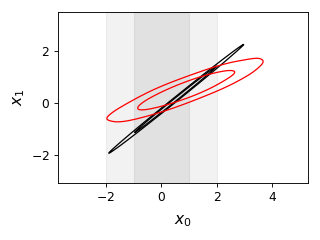`add_x_marker`(marker, color=None, ls=None, lw=None, ax=None, **kwargs)[source]

Adds a vertical line marking some x value. Optional arguments can override default settings.

Parameters: marker – The x coordinate of the location the marker line color – optional color of the marker ls – optional line style of the marker lw – optional line width ax – optional `Axes` instance (or y,x subplot coordinate) to add to (defaults to current plot or the first/main plot if none) kwargs – additional arguments to pass to `axvline()`
`add_y_bands`(y, sigma, color='gray', ax=None, alpha1=0.15, alpha2=0.1, **kwargs)[source]

Parameters: y – central y value for bands sigma – 1 sigma error on y color – The base color to use ax – optional `Axes` instance (or y,x subplot coordinate) to add to (defaults to current plot or the first/main plot if none) alpha1 – alpha for the 1 sigma band; note this is drawn on top of the 2 sigma band. Set to zero if you only want 2 sigma band alpha2 – alpha for the 2 sigma band. Set to zero if you only want 1 sigma band kwargs – optional keyword arguments for `axhspan()`
```from getdist import plots, gaussian_mixtures
samples = gaussian_mixtures.randomTestMCSamples(ndim=2, nMCSamples=1)
g = plots.get_single_plotter(width_inch=4)
g.plot_2d(samples, ['x0','x1'], filled=True);
```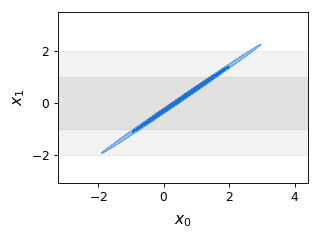`add_y_marker`(marker, color=None, ls=None, lw=None, ax=None, **kwargs)[source]

Adds a horizontal line marking some y value. Optional arguments can override default settings.

Parameters: marker – The y coordinate of the location the marker line color – optional color of the marker ls – optional line style of the marker lw – optional line width. ax – optional `Axes` instance (or y,x subplot coordinate) to add to (defaults to current plot or the first/main plot if none) kwargs – additional arguments to pass to `axhline()`
`default_col_row`(nplot=1, nx=None, ny=None)[source]

Get default subplot columns and rows depending on number of subplots.

Parameters: nplot – total number of subplots nx – optional specified number of columns ny – optional specified number of rows n_cols, n_rows
`export`(fname=None, adir=None, watermark=None, tag=None)[source]

Exports given figure to a file. If the filename is not specified, saves to a file with the same name as the calling script (useful for plot scripts where the script name matches the output figure).

Parameters: fname – The filename to export to. The extension (.pdf, .png, etc) determines the file type adir – The directory to save to watermark – a watermark text, e.g. to make the plot with some pre-final version number tag – A suffix to add to the filename.
`finish_plot`(legend_labels=None, legend_loc=None, line_offset=0, legend_ncol=None, label_order=None, no_extra_legend_space=False, no_tight=False, **legend_args)[source]

Finish the current plot, adjusting subplot spacing and adding legend if required.

Parameters: legend_labels – The labels for a figure legend legend_loc – The legend location, default from settings (figure_legend_loc) line_offset – The offset of plotted lines to label (e.g. 1 to not label first line) legend_ncol – The number of columns in the legend, defaults to 1 label_order – minus one to show legends in reverse order that lines were added, or a list giving specific order of line indices no_extra_legend_space – True to put figure legend inside the figure box no_tight – don’t use `tight_layout()` to adjust subplot positions legend_args – optional parameters for the legend
`get_axes`(ax=None, pars=None)[source]

Get the axes instance corresponding to the given subplot (y,x) coordinates, parameter list, or otherwise if ax is None get the last subplot axes used, or generate the first (possibly only) subplot if none.

Parameters: ax – optional `Axes`, (y,x) subplot coordinate, tuple of parameter names, or None to get last axes used or otherwise default to first subplot pars – optional list of parameters to associate with the axes an `Axes` instance, or None if the specified axes don’t exist
`get_axes_for_params`(*pars, **kwargs)[source]

Get axes corresponding to given parameters

Parameters: pars – x or x,y or x,y,color parameters kwargs – set ordered=False to match y,x as well as x,y axes instance or None if not found
`get_param_array`(root, params: Union[None, str, Sequence[T_co]] = None, renames: Mapping[KT, VT_co] = None)[source]

Gets an array of `ParamInfo` for named params in the given root.

If a parameter is not found in root, returns the original ParamInfo if ParamInfo was passed, or fails otherwise.

Parameters: root – The root name of the samples to use params – the parameter names (if not specified, get all) renames – optional dictionary mapping input names and equivalent names used by the samples list of `ParamInfo` instances for the parameters
`make_figure`(nplot=1, nx=None, ny=None, xstretch=1.0, ystretch=1.0, sharex=False, sharey=False)[source]

Makes a new figure with one or more subplots.

Parameters: nplot – number of subplots nx – number of subplots in each row ny – number of subplots in each column xstretch – The parameter of how much to stretch the width, 1 is default ystretch – The parameter of how much to stretch the height, 1 is default. Note this multiplies settings.subplot_size_ratio before determining actual stretch. sharex – no vertical space between subplots sharey – no horizontal space between subplots The plot_col, plot_row numbers of subplots for the figure
`new_plot`(close_existing=None)[source]

Resets the given plotter to make a new empty plot.

Parameters: close_existing – True to close any current figure
`param_bounds_for_root`(root)[source]

Get any hard prior bounds for the parameters with root file name

Parameters: root – The root name to be used object with get_upper() or getUpper() and get_lower() or getLower() bounds functions
`param_latex_label`(root, name, label_params=None)[source]

Returns the latex label for given parameter.

Parameters: root – root name of the samples having the parameter (or `MCSamples` instance) name – The param name label_params – optional name of .paramnames file to override parameter name labels The latex label
`param_names_for_root`(root)[source]

Get the parameter names and labels `ParamNames` instance for the given root name

Parameters: root – The root name of the samples. `ParamNames` instance
`plot_1d`(roots, param, marker=None, marker_color=None, label_right=False, title_limit=None, no_ylabel=False, no_ytick=False, no_zero=False, normalized=False, param_renames=None, ax=None, **kwargs)[source]

Make a single 1D plot with marginalized density lines.

Parameters: roots – root name or `MCSamples` instance (or list of any of either of these) for the samples to plot param – the parameter name to plot marker – If set, places a marker at given coordinate. marker_color – If set, sets the marker color. label_right – If True, label the y axis on the right rather than the left title_limit – If not None, a maginalized limit (1,2..) of the first root to print as the title of the plot no_ylabel – If True excludes the label on the y axis no_ytick – If True show no y ticks no_zero – If true does not show tick label at zero on y axis normalized – plot normalized densities (if False, densities normalized to peak at 1) param_renames – optional dictionary mapping input parameter names to equivalent names used by the samples ax – optional `Axes` instance (or y,x subplot coordinate) to add to (defaults to current plot or the first/main plot if none) kwargs – additional optional keyword arguments: lims: optional limits for x range of the plot [xmin, xmax] ls : list of line styles for the different lines plotted colors: list of colors for the different lines plotted lws: list of line widths for the different lines plotted alphas: list of alphas for the different lines plotted line_args: a list of dictionaries with settings for each set of lines arguments for `set_axes()`
```from getdist import plots, gaussian_mixtures
samples1, samples2 = gaussian_mixtures.randomTestMCSamples(ndim=2, nMCSamples=2)
g = plots.get_single_plotter(width_inch=4)
g.plot_1d([samples1, samples2], 'x0', marker=0)
```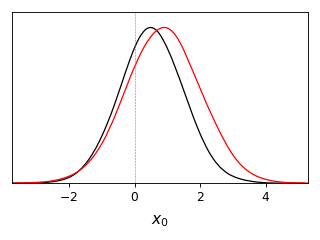```from getdist import plots, gaussian_mixtures
samples1, samples2 = gaussian_mixtures.randomTestMCSamples(ndim=2, nMCSamples=2)
g = plots.get_single_plotter(width_inch=3)
g.plot_1d([samples1, samples2], 'x0', normalized=True, colors=['green','black'])
```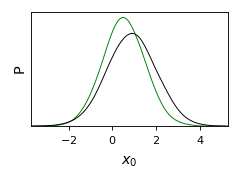`plot_2d`(roots, param1=None, param2=None, param_pair=None, shaded=False, add_legend_proxy=True, line_offset=0, proxy_root_exclude=(), ax=None, **kwargs)[source]

Create a single 2D line, contour or filled plot.

Parameters: roots – root name or `MCSamples` instance (or list of any of either of these) for the samples to plot param1 – x parameter name param2 – y parameter name param_pair – An [x,y] pair of params; can be set instead of param1 and param2 shaded – True if plot should be a shaded density plot (for the first samples plotted) add_legend_proxy – True if should add to the legend proxy line_offset – line_offset if not adding first contours to plot proxy_root_exclude – any root names not to include when adding to the legend proxy ax – optional `Axes` instance (or y,x subplot coordinate) to add to (defaults to current plot or the first/main plot if none) kwargs – additional optional arguments: filled: True for filled contours lims: list of limits for the plot [xmin, xmax, ymin, ymax] ls : list of line styles for the different sample contours plotted colors: list of colors for the different sample contours plotted lws: list of line widths for the different sample contours plotted alphas: list of alphas for the different sample contours plotted line_args: a list of dictionaries with settings for each set of contours arguments for `set_axes()` The xbounds, ybounds of the plot.
```from getdist import plots, gaussian_mixtures
samples1, samples2 = gaussian_mixtures.randomTestMCSamples(ndim=4, nMCSamples=2)
g = plots.get_single_plotter(width_inch = 4)
g.plot_2d([samples1,samples2], 'x1', 'x2', filled=True);
```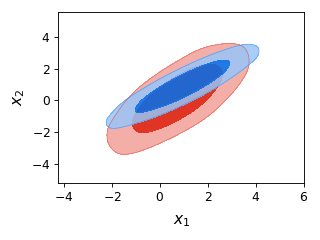`plot_2d_scatter`(roots, param1, param2, color='k', line_offset=0, add_legend_proxy=True, **kwargs)[source]

Make a 2D sample scatter plot.

If roots is a list of more than one, additional densities are plotted as contour lines.

Parameters: roots – root name or `MCSamples` instance (or list of any of either of these) for the samples to plot param1 – name of x parameter param2 – name of y parameter color – color to plot the samples line_offset – The line index offset for added contours add_legend_proxy – True if should add a legend proxy kwargs – additional optional arguments: filled: True for filled contours for second and later items in roots lims: limits for the plot [xmin, xmax, ymin, ymax] ls : list of line styles for the different sample contours plotted colors: list of colors for the different sample contours plotted lws: list of linewidths for the different sample contours plotted alphas: list of alphas for the different sample contours plotted line_args: a list of dict with settings for contours from each root
`plot_3d`(roots, params=None, params_for_plots=None, color_bar=True, line_offset=0, add_legend_proxy=True, alpha_samples=False, ax=None, **kwargs)[source]

Make a 2D scatter plot colored by the value of a third parameter (a 3D plot).

If roots is a list of more than one, additional densities are plotted as contour lines.

Parameters: roots – root name or `MCSamples` instance (or list of any of either of these) for the samples to plot params – list with the three parameter names to plot (x, y, color) params_for_plots – list of parameter triplets to plot for each root plotted; more general alternative to params color_bar – True if should include a color bar line_offset – The line index offset for added contours add_legend_proxy – True if should add a legend proxy alpha_samples – if True, use alternative scatter style where all samples are plotted alphaed by their weights ax – optional `Axes` instance (or y,x subplot coordinate) to add to (defaults to current plot or the first/main plot if none) kwargs – additional optional arguments: filled: True for filled contours for second and later items in roots lims: limits for the plot [xmin, xmax, ymin, ymax] ls : list of line styles for the different sample contours plotted colors: list of colors for the different sample contours plotted lws: list of linewidths for the different sample contours plotted alphas: list of alphas for the different sample contours plotted line_args: a list of dict with settings for contours from each root arguments for `add_colorbar()`
```from getdist import plots, gaussian_mixtures
samples1, samples2 = gaussian_mixtures.randomTestMCSamples(ndim=3, nMCSamples=2)
g = plots.get_single_plotter(width_inch=4)
g.plot_3d([samples1, samples2], ['x0','x1','x2']);
```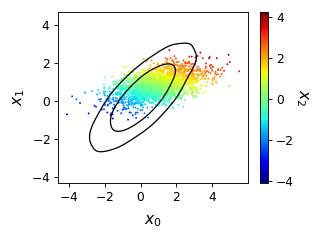`plot_4d`(roots, params, color_bar=True, colorbar_args: Mapping[KT, VT_co] = mappingproxy({}), ax=None, lims=mappingproxy({}), azim: Optional[float] = 15, elev: Optional[float] = None, dist: float = 12, alpha: Union[float, Sequence[float]] = 0.5, marker='o', max_scatter_points: Optional[int] = None, shadow_color=None, shadow_alpha=0.1, fixed_color=None, compare_colors=None, animate=False, anim_angle_degrees=360, anim_step_degrees=0.6, anim_fps=15, mp4_filename: Optional[str] = None, mp4_bitrate=-1, **kwargs)[source]

Make a 3d x-y-z scatter plot colored by the value of a fourth parameter. If animate is True, it will rotate, and can be saved to an mp4 video file by setting mp4_filename (you must have ffmpeg installed). Note animations can be quite slow to render.

Parameters: roots – root name or `MCSamples` instance (or list of any of either of these) for the samples to plot params – list with the three parameter names to plot and color (x, y, x, color); can also set fixed_color and specify just three parameters color_bar – True if should include a color bar colorbar_args – extra arguments for colorbar ax – optional `Axes3D` instance to add to (defaults to current plot or the first/main plot if none) lims – dictionary of optional limits, e.g. {‘param1’:(min1, max1),’param2’:(min2,max2)}. If this include parameters that are not plotted, the samples outside the limits will still be removed azim – azimuth for initial view elev – elevation for initial view dist – distance for view (make larger if labels out of area) alpha – alpha, or list of alphas for each root, to use for scatter samples marker – marker, or list of markers for each root max_scatter_points – if set, maximum number of points to plots from each root shadow_color – if not None, a color value (or list of color values) to use for plotting axes-projected samples; or True to plot gray shadows shadow_alpha – if not None, separate alpha or list of alpha for shadows fixed_color – if not None, a fixed color for the the first-root scatter plot rather than a 4th parameter value compare_colors – if not None, fixed scatter color for second-and-higher roots rather than using 4th parameter value animate – if True, rotate the plot anim_angle_degrees – total angle for animation rotation anim_step_degrees – angle per frame anim_fps – animation frames per second mp4_filename – if animating, optional filename to produce mp4 video mp4_bitrate – bitrate kwargs – additional optional arguments for `scatter()`
```from getdist import plots, gaussian_mixtures

samples1, samples2 = gaussian_mixtures.randomTestMCSamples(ndim=4, nMCSamples=2)
samples1.samples[:, 0] *= 5  # stretch out in one direction
g = plots.get_single_plotter()
g.plot_4d([samples1, samples2], ['x0', 'x1', 'x2', 'x3'],
cmap='viridis', color_bar=False,
```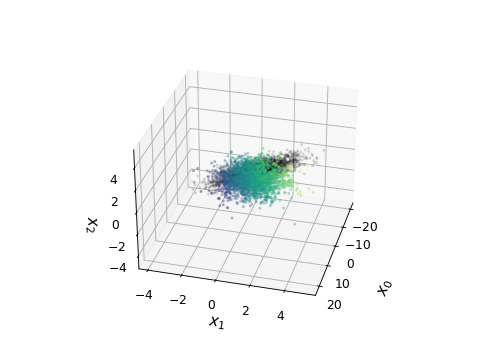```from getdist import plots, gaussian_mixtures

samples1 = gaussian_mixtures.randomTestMCSamples(ndim=4)
samples1.samples[:, 0] *= 5  # stretch out in one direction
g = plots.get_single_plotter()
g.plot_4d(samples1, ['x0', 'x1', 'x2', 'x3'], cmap='jet',
max_scatter_points=6000,
lims={'x2': (-3, 3), 'x3': (-3, 3)},
colorbar_args={'shrink': 0.6})
```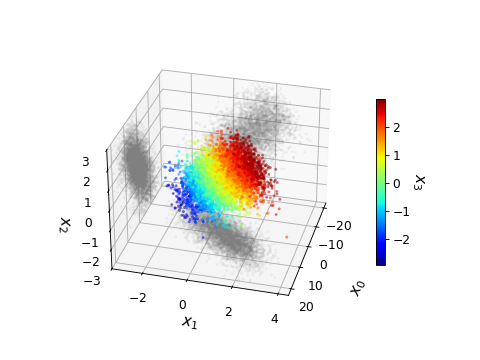Generate an mp4 video (in jupyter, using a notebook rather than inline matplotlib):

```g.plot_4d([samples1, samples2], ['x0', 'x1', 'x2', 'x3'], cmap='viridis',
compare_colors=['k'],
animate=True, mp4_filename='sample_rotation.mp4', mp4_bitrate=1024, anim_fps=20)
```
`plots_1d`(roots, params=None, legend_labels=None, legend_ncol=None, label_order=None, nx=None, param_list=None, roots_per_param=False, share_y=None, markers=None, title_limit=None, xlims=None, param_renames=None, **kwargs)[source]

Make an array of 1D marginalized density subplots

Parameters: roots – root name or `MCSamples` instance (or list of any of either of these) for the samples to plot params – list of names of parameters to plot legend_labels – list of legend labels legend_ncol – Number of columns for the legend. label_order – minus one to show legends in reverse order that lines were added, or a list giving specific order of line indices nx – number of subplots per row param_list – name of .paramnames file listing specific subset of parameters to plot roots_per_param – True to use a different set of samples for each parameter: plots param[i] using roots[i] (where roots[i] is the list of sample root names to use for plotting parameter i). This is useful for example for plotting one-parameter extensions of a baseline model, each with various data combinations. share_y – True for subplots to share a common y axis with no horizontal space between subplots markers – optional dict giving vertical marker values indexed by parameter, or a list of marker values for each parameter plotted title_limit – if not None, a maginalized limit (1,2..) of the first root to print as the title of each of the plots xlims – list of [min,max] limits for the range of each parameter plot param_renames – optional dictionary holding mapping between input names and equivalent names used in the samples. kwargs – optional keyword arguments for `plot_1d()` The plot_col, plot_row subplot dimensions of the new figure
```from getdist import plots, gaussian_mixtures
samples1, samples2 = gaussian_mixtures.randomTestMCSamples(ndim=4, nMCSamples=2)
g = plots.get_subplot_plotter()
g.plots_1d([samples1, samples2], ['x0', 'x1', 'x2'], nx=3, share_y=True, legend_ncol =2,
markers={'x1':0}, colors=['red', 'green'], ls=['--', '-.'])
```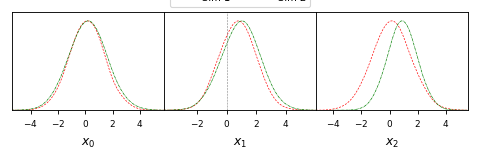`plots_2d`(roots, param1=None, params2=None, param_pairs=None, nx=None, legend_labels=None, legend_ncol=None, label_order=None, filled=False, shaded=False, **kwargs)[source]

Make an array of 2D line, filled or contour plots.

Parameters: roots – root name or `MCSamples` instance (or list of either of these) for the samples to plot param1 – x parameter to plot params2 – list of y parameters to plot against x param_pairs – list of [x,y] parameter pairs to plot; either specify param1, param2, or param_pairs nx – number of subplots per row legend_labels – The labels used for the legend. legend_ncol – The amount of columns in the legend. label_order – minus one to show legends in reverse order that lines were added, or a list giving specific order of line indices filled – True to plot filled contours shaded – True to shade by the density for the first root plotted kwargs – optional keyword arguments for `plot_2d()` The plot_col, plot_row subplot dimensions of the new figure
```from getdist import plots, gaussian_mixtures
samples1, samples2 = gaussian_mixtures.randomTestMCSamples(ndim=4, nMCSamples=2)
g = plots.get_subplot_plotter(subplot_size=4)
g.settings.legend_frac_subplot_margin = 0.05
g.plots_2d([samples1, samples2], param_pairs=[['x0', 'x1'], ['x1', 'x2']],
nx=2, legend_ncol=2, colors=['blue', 'red'])
```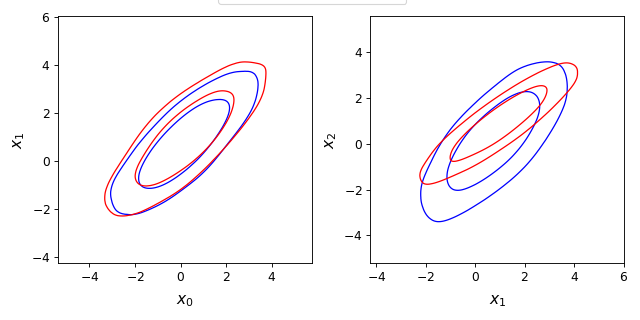`plots_2d_triplets`(root_params_triplets, nx=None, filled=False, x_lim=None)[source]

Creates an array of 2D plots, where each plot uses different samples, x and y parameters

Parameters: root_params_triplets – a list of (root, x, y) giving sample root names, and x and y parameter names to plot in each subplot nx – number of subplots per row filled – True for filled contours x_lim – limits for all the x axes. The plot_col, plot_row subplot dimensions of the new figure
`plots_3d`(roots, param_sets, nx=None, legend_labels=None, **kwargs)[source]

Create multiple 3D subplots

Parameters: roots – root name or `MCSamples` instance (or list of any of either of these) for the samples to plot param_sets – A list of triplets of parameter names to plot [(x,y, color), (x2,y2,color2)..] nx – number of subplots per row legend_labels – list of legend labels kwargs – keyword arguments for `plot_3d()` The plot_col, plot_row subplot dimensions of the new figure
```from getdist import plots, gaussian_mixtures
samples1, samples2 = gaussian_mixtures.randomTestMCSamples(ndim=5, nMCSamples=2)
g = plots.get_subplot_plotter(subplot_size=4)
g.plots_3d([samples1, samples2], [['x0', 'x1', 'x2'], ['x3', 'x4', 'x2']], nx=2);
````plots_3d_z`(roots, param_x, param_y, param_z=None, max_z=None, **kwargs)[source]

Make set of sample scatter subplots of param_x against param_y, each coloured by values of parameters in param_z (all if None). Any second or more samples in root are shown as contours.

Parameters: roots – root name or `MCSamples` instance (or list of any of either of these) for the samples to plot param_x – x parameter name param_y – y parameter name param_z – list of parameter to names to color samples in each subplot (default: all) max_z – The maximum number of z parameters we should use. kwargs – keyword arguments for `plot_3d()` The plot_col, plot_row subplot dimensions of the new figure
`rectangle_plot`(xparams, yparams, yroots=None, roots=None, plot_roots=None, plot_texts=None, xmarkers=None, ymarkers=None, marker_args=mappingproxy({}), param_limits=mappingproxy({}), legend_labels=None, legend_ncol=None, label_order=None, **kwargs)[source]

Make a grid of 2D plots.

A rectangle plot shows all x parameters plotted againts all y parameters in a grid of subplots with no spacing.

Set roots to use the same set of roots for every plot in the rectangle, or set yroots (list of list of roots) to use different set of roots for each row of the plot; alternatively plot_roots allows you to specify explicitly (via list of list of list of roots) the set of roots for each individual subplot.

Parameters: xparams – list of parameters for the x axes yparams – list of parameters for the y axes yroots – (list of list of roots) allows use of different set of root names for each row of the plot; set either roots or yroots roots – list of root names or `MCSamples` instances. Uses the same set of roots for every plot in the rectangle; set either roots or yroots. plot_roots – Allows you to specify (via list of list of list of roots) the set of roots for each individual subplot plot_texts – a 2D array (or list of lists) of a text label to put in each subplot (use a None entry to skip one) xmarkers – optional dict giving vertical marker values indexed by parameter, or a list of marker values for each x parameter plotted ymarkers – optional dict giving horizontal marker values indexed by parameter, or a list of marker values for each y parameter plotted marker_args – arguments for `add_x_marker()` and `add_y_marker()` param_limits – a dictionary holding a mapping from parameter names to axis limits for that parameter legend_labels – list of labels for the legend legend_ncol – The number of columns for the legend label_order – minus one to show legends in reverse order that lines were added, or a list giving specific order of line indices kwargs – arguments for `plot_2d()`. the 2D list of `Axes` created
```from getdist import plots, gaussian_mixtures
samples1, samples2 = gaussian_mixtures.randomTestMCSamples(ndim=4, nMCSamples=2)
g = plots.get_subplot_plotter()
g.rectangle_plot(['x0','x1'], ['x2','x3'], roots = [samples1, samples2], filled=True)
```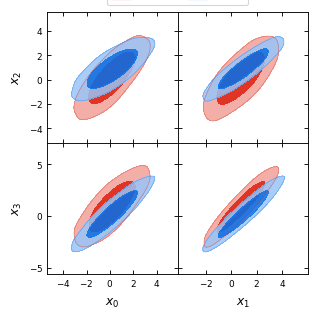`rotate_xticklabels`(ax=None, rotation=90, labelsize=None)[source]

Rotates the x-tick labels by given rotation (degrees)

Parameters: ax – optional `Axes` instance (or y,x subplot coordinate) to add to (defaults to current plot or the first/main plot if none) rotation – How much to rotate in degrees. labelsize – size for tick labels (default from settings.axes_fontsize)
`rotate_yticklabels`(ax=None, rotation=90, labelsize=None)[source]

Rotates the y-tick labels by given rotation (degrees)

Parameters: ax – optional `Axes` instance (or y,x subplot coordinate) to add to (defaults to current plot or the first/main plot if none) rotation – How much to rotate in degrees. labelsize – size for tick labels (default from settings.axes_fontsize)
`samples_for_root`(root, file_root=None, cache=True, settings=None)[source]

Gets `MCSamples` from root name (or just return root if it is already an MCSamples instance).

Parameters: root – The root name (without path, e.g. my_chains) file_root – optional full root path, by default searches in self.chain_dirs cache – if True, return cached object if already loaded settings – optional dictionary of settings to use `MCSamples` for the given root name
`set_axes`(params=(), lims=None, do_xlabel=True, do_ylabel=True, no_label_no_numbers=False, pos=None, color_label_in_axes=False, ax=None, **_other_args)[source]

Set the axis labels and ticks, and various styles. Do not usually need to call this directly.

Parameters: params – [x,y] list of the `ParamInfo` for the x and y parameters to use for labels lims – optional [xmin, xmax, ymin, ymax] to fix specific limits for the axes do_xlabel – True if should include label for x axis. do_ylabel – True if should include label for y axis. no_label_no_numbers – True to hide tick labels pos – optional position of the axes [‘left’ | ‘bottom’ | ‘width’ | ‘height’] color_label_in_axes – If True, and params has at last three entries, puts text in the axis to label the third parameter ax – optional `Axes` instance (or y,x subplot coordinate) to add to (defaults to current plot or the first/main plot if none) _other_args – Not used, just quietly ignore so that set_axes can be passed general kwargs an `Axes` instance
`set_xlabel`(param, ax=None)[source]

Sets the label for the x axis.

Parameters: param – the `ParamInfo` for the x axis parameter ax – optional `Axes` instance (or y,x subplot coordinate) to add to (defaults to current plot or the first/main plot if none)
`set_ylabel`(param, ax=None, **kwargs)[source]

Sets the label for the y axis.

Parameters: param – the `ParamInfo` for the y axis parameter ax – optional `Axes` instance (or y,x subplot coordinate) to add to (defaults to current plot or the first/main plot if none) kwargs – optional extra arguments for Axes set_ylabel
`set_zlabel`(param, ax=None, **kwargs)[source]

Sets the label for the z axis.

Parameters: param – the `ParamInfo` for the y axis parameter ax – optional `Axes` instance (or y,x subplot coordinate) to add to (defaults to current plot or the first/main plot if none) kwargs – optional extra arguments for Axes set_zlabel
`show_all_settings`()[source]

Prints settings and library versions

`triangle_plot`(roots, params=None, legend_labels=None, plot_3d_with_param=None, filled=False, shaded=False, contour_args=None, contour_colors=None, contour_ls=None, contour_lws=None, line_args=None, label_order=None, legend_ncol=None, legend_loc=None, title_limit=None, upper_roots=None, upper_kwargs=mappingproxy({}), upper_label_right=False, diag1d_kwargs=mappingproxy({}), markers=None, marker_args=mappingproxy({}), param_limits=mappingproxy({}), **kwargs)[source]

Make a trianglular array of 1D and 2D plots.

A triangle plot is an array of subplots with 1D plots along the diagonal, and 2D plots in the lower corner. The upper triangle can also be used by setting upper_roots.

Parameters: roots – root name or `MCSamples` instance (or list of any of either of these) for the samples to plot params – list of parameters to plot (default: all, can also use glob patterns to match groups of parameters) legend_labels – list of legend labels plot_3d_with_param – for the 2D plots, make sample scatter plot, with samples colored by this parameter name (to make a ‘3D’ plot) filled – True for filled contours shaded – plot shaded density for first root (cannot be used with filled) contour_args – optional dict (or list of dict) with arguments for each 2D plot (e.g. specifying color, alpha, etc) contour_colors – list of colors for plotting contours (for each root) contour_ls – list of Line styles for contours (for each root) contour_lws – list of Line widths for contours (for each root) line_args – dict (or list of dict) with arguments for each 2D plot (e.g. specifying ls, lw, color, etc) label_order – minus one to show legends in reverse order that lines were added, or a list giving specific order of line indices legend_ncol – The number of columns for the legend legend_loc – The location for the legend title_limit – if not None, a maginalized limit (1,2..) to print as the title of the first root on the diagonal 1D plots upper_roots – set to fill the upper triangle with subplots using this list of sample root names upper_kwargs – dict for same-named arguments for use when making upper-triangle 2D plots (contour_colors, etc). Set show_1d=False to not add to the diagonal. upper_label_right – when using upper_roots whether to label the y axis on the top-right axes (splits labels between left and right, but avoids labelling 1D y axes top left) diag1d_kwargs – list of dict for arguments when making 1D plots on grid diagonal markers – optional dict giving marker values indexed by parameter, or a list of marker values for each parameter plotted marker_args – dictionary of optional arguments for adding markers (passed to axvline and/or axhline) param_limits – a dictionary holding a mapping from parameter names to axis limits for that parameter kwargs – optional keyword arguments for `plot_2d()` or `plot_3d()` (lower triangle only)
```from getdist import plots, gaussian_mixtures
samples1, samples2 = gaussian_mixtures.randomTestMCSamples(ndim=4, nMCSamples=2)
g = plots.get_subplot_plotter()
g.triangle_plot([samples1, samples2], filled=True, legend_labels = ['Contour 1', 'Contour 2'])
```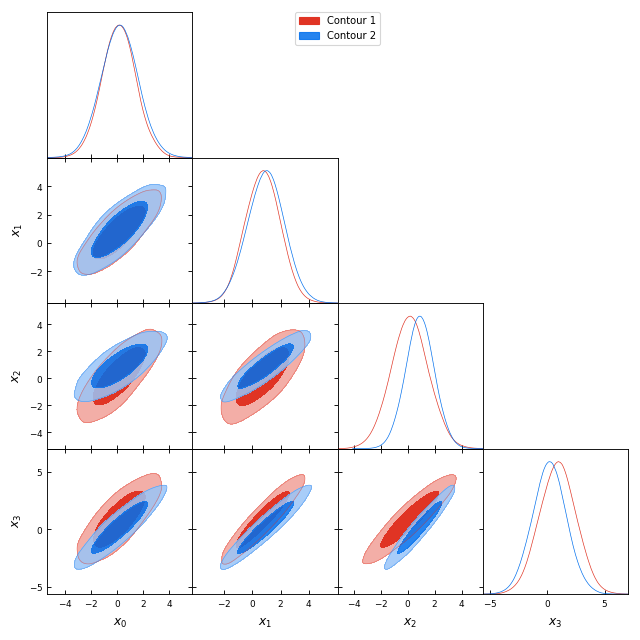```from getdist import plots, gaussian_mixtures
samples1, samples2 = gaussian_mixtures.randomTestMCSamples(ndim=4, nMCSamples=2)
g = plots.get_subplot_plotter()
g.triangle_plot([samples1, samples2], ['x0','x1','x2'], plot_3d_with_param='x3')
```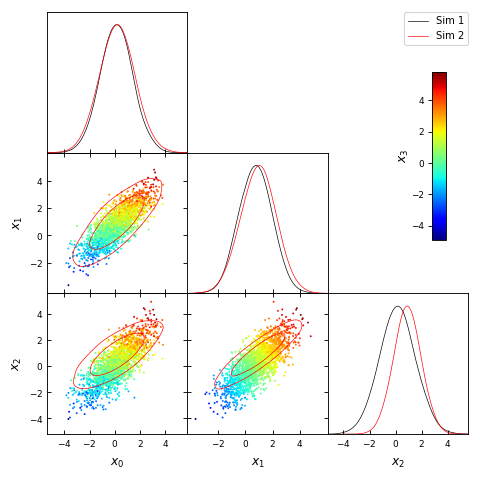class `getdist.plots.``MCSampleAnalysis`(chain_locations: Union[str, Iterable[str]], settings: Union[str, dict, getdist.inifile.IniFile] = None)[source]

A class that loads and analyses samples, mapping root names to `MCSamples` objects with caching. Typically accessed as the instance stored in plotter.sample_analyser, for example to get an `MCSamples` instance from a root name being used by a plotter, use plotter.sample_analyser.samples_for_root(name).

Parameters: chain_locations – either a directory or the path of a grid of runs; it can also be a list of such, which is searched in order settings – Either an `IniFile` instance, the name of an .ini file, or a dict holding sample analysis settings.
`add_chain_dir`(chain_dir)[source]

Adds a new chain directory or grid path for searching for samples

Parameters: chain_dir – The root directory to add
`add_root`(file_root)[source]

Add a root file for some new samples

Parameters: file_root – Either a file root name including path or a `RootInfo` instance `MCSamples` instance for given root file.
`add_roots`(roots)[source]

Parameters: roots – An iterable containing filenames or `RootInfo` objects to add
`bounds_for_root`(root)[source]

Returns an object with get_upper/getUpper and get_lower/getLower to get hard prior bounds for given root name

Parameters: root – The root name to use. object with get_upper() or getUpper() and get_lower() or getLower() functions
`get_density`(root, param, likes=False)[source]

Get `Density1D` for given root name and parameter

Parameters: root – The root name of the samples to use param – name of the parameter likes – whether to include mean likelihood in density.likes `Density1D` instance with 1D marginalized density
`get_density_grid`(root, param1, param2, conts=2, likes=False)[source]

Get 2D marginalized density for given root name and parameters

Parameters: root – The root name for samples to use. param1 – x parameter param2 – y parameter conts – number of contour levels (up to maximum calculated using contours in analysis settings) likes – whether to include mean likelihoods `Density2D` instance with marginalized density
`load_single_samples`(root)[source]

Gets a set of unit weight samples for given root name, e.g. for making sample scatter plot

Parameters: root – The root name to use. array of unit weight samples
`params_for_root`(root, label_params=None)[source]

Returns a `ParamNames` with names and labels for parameters used by samples with a given root name.

Parameters: root – The root name of the samples to use. label_params – optional name of .paramnames file containing labels to use for plots, overriding default `ParamNames` instance
`remove_root`(root)[source]

Remove a given root file (does not delete it)

Parameters: root – The root name to remove
`reset`(settings=None, chain_settings_have_priority=True)[source]

Resets the caches, starting afresh optionally with new analysis settings

Parameters: settings – Either an `IniFile` instance, the name of an .ini file, or a dict holding sample analysis settings. chain_settings_have_priority – whether to prioritize settings saved with the chain
`samples_for_root`(root: Union[str, getdist.mcsamples.MCSamples], file_root: Optional[str] = None, cache=True, settings: Optional[Mapping[str, Any]] = None)[source]

Gets `MCSamples` from root name (or just return root if it is already an MCSamples instance).

Parameters: root – The root name (without path, e.g. my_chains) file_root – optional full root path, by default searches in self.chain_dirs cache – if True, return cached object if already loaded settings – optional dictionary of settings to use `MCSamples` for the given root name
`getdist.plots.``add_plotter_style`(name, cls, activate=False)[source]

Add a plotting style, consistenting of style name and a class type to use when making plotter instances.

Parameters: name – name for the style cls – a class inherited from `GetDistPlotter` activate – whether to make it the active style
`getdist.plots.``get_plotter`(style: Optional[str] = None, **kwargs)[source]

Creates a new plotter and returns it

Parameters: style – name of a plotter style (associated with custom plotter class/settings), otherwise uses active kwargs – arguments for the style’s `GetDistPlotter` The `GetDistPlotter` instance
`getdist.plots.``get_single_plotter`(ratio: Optional[float] = None, width_inch: Optional[float] = None, scaling: Optional[bool] = None, rc_sizes=False, style: Optional[str] = None, **kwargs)[source]

Get a `GetDistPlotter` for making a single plot of fixed width.

For a half-column plot for a paper use width_inch=3.464.

Use this or `get_subplot_plotter()` to make a `GetDistPlotter` instance for making plots. This function will use the active style by default, which will determine defaults for the various optional parameters (see `set_active_style()`).

Parameters: ratio – The ratio between height and width. width_inch – The width of the plot in inches scaling – whether to scale down fonts and line widths for small subplot axis sizes (relative to reference sizes, 3.5 inch) rc_sizes – set default font sizes from matplotlib’s current rcParams if no explicit settings passed in kwargs style – name of a plotter style (associated with custom plotter class/settings), otherwise uses active kwargs – arguments for `GetDistPlotter` The `GetDistPlotter` instance
`getdist.plots.``get_subplot_plotter`(subplot_size: Optional[float] = None, width_inch: Optional[float] = None, scaling: Optional[bool] = None, rc_sizes=False, subplot_size_ratio: Optional[float] = None, style: Optional[str] = None, **kwargs) → getdist.plots.GetDistPlotter[source]

Get a `GetDistPlotter` for making an array of subplots.

If width_inch is None, just makes plot as big as needed for given subplot_size, otherwise fixes total width and sets default font sizes etc. from matplotlib’s default rcParams.

Use this or `get_single_plotter()` to make a `GetDistPlotter` instance for making plots. This function will use the active style by default, which will determine defaults for the various optional parameters (see `set_active_style()`).

Parameters: subplot_size – The size of each subplot in inches width_inch – Optional total width in inches scaling – whether to scale down fonts and line widths for small sizes (relative to reference sizes, 3.5 inch) rc_sizes – set default font sizes from matplotlib’s current rcParams if no explicit settings passed in kwargs subplot_size_ratio – ratio of height to width for subplots style – name of a plotter style (associated with custom plotter class/settings), otherwise uses active kwargs – arguments for `GetDistPlotter` The `GetDistPlotter` instance
`getdist.plots.``set_active_style`(name=None)[source]

Set an active style name. Each style name is associated with a `GetDistPlotter` class used to generate plots, with optional custom plot settings and rcParams. The corresponding style module must have been loaded before using this.

Note that because style modules can change rcParams, which is a global parameter, in general style settings are changed globally until changed back. But if your style does not change rcParams then you can also just pass a style name parameter when you make a plot instance.

The supplied example styles are ‘default’, ‘tab10’ (default matplotlib color scheme) and ‘planck’ (more compilcated example using latex and various customized settings). Use `add_plotter_style()` to add your own style class.

Parameters: name – name of the style, or none to revert to default the previously active style name